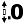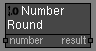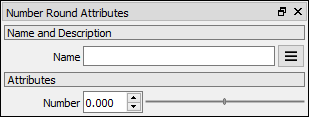# Number Round node

Left Toolbar >Math Nodes >Number Round

The Number Round node returns the value of a number rounded to the nearest integer.

## Procedure

### To calculate the round of the number in the node graph:

1. On the left toolbar choose Math Nodes> Number Round2. Drag the Number Round icon into the Node Graph.3. Connect the Output: result.

## Inputs

number A NUMBER type of value.

## Outputs

Result The number value.

## Attributes PanelNumber Number value. This attribute is exposed as input by default.

Math Nodes

Main Page